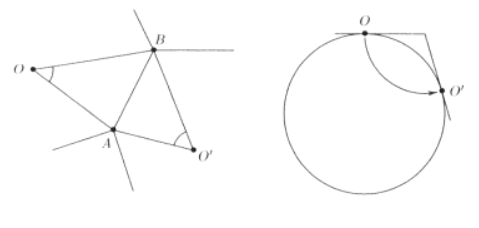# Polyhedron not circumscribed about a sphere

Let $P$ be a polyhedron whose faces are colored black and white so that there are more black faces and no two black faces are adjacent. Show that $P$ is not circumscribed about a sphere.

My teacher has conjectured this. I haven't been able to produce a counterexample, neither a solution. Is this claim true? If not a counterexample would be nice. Thanks in advance for helping.

• One way to get examples of polyhedra with more black faces than white, no two adjacent, is to start with a polyhedron with more vertices than faces, then truncate it, coloring the new faces black and the old faces white. So far the examples like this that I have tried can't be circumscribed about a sphere. – Douglas Zare Jun 23 '14 at 11:46
• The polar dual of a circumscribable polyhedron is inscribable. Perhaps the work on conditions for inscribable polyhedra can help: Michael Dillencourt and Warren Smith. "Graph-theoretical conditions for inscribability and Delaunay realizability." Discrete Mathematics 161.1 (1996): 63-77. – Joseph O'Rourke Jun 23 '14 at 11:52

This is exercise 21.3, of Mathematical Omnibus: Thirty Lectures on Classic Mathematics, by D.B. Fuks and Serge Tabachnikov. The solution is given on page 448:

Assume that $$P$$ is circumscribed. Consider a face $$A_1$$, $$A_2$$,... $$A_n$$ and let $$O$$ be its tangency point with the sphere. Clearly, the sum of angles $$A_i O A_{i+1}$$ is $$2\pi$$. We shall sum up al these angles over all faces, taking the angles in the white faces with positive signs and the angles in the black faces with negative signs. Since there are more black faces, this sum, $$\Sigma$$, is negative.

On the other hand, consider two adjacent faces with a common edge $$AB$$; see figure; The angles $$AOB$$ and $$AO'B$$ are equal. Indeed, revolve the plane $$AOB$$ about the line $$AB$$ (as if it were a hinge) until it coincides with the plane $$AO'B$$. This rotation takes point $$O$$ to $$O'$$ and hence the triangles $$AOB$$ and $$AO'B$$ are congruent.

There are two kinds of adjacent faces; black-white and white-white; the former's contribution to $$\Sigma$$ cancels, and the latter contribute a positive number. Thus $$\Sigma\geq 0$$, a contradiction.• Very impressive. I may just have to buy this book. – Todd Trimble Jun 23 '14 at 12:40
• I have a question, how can we ensure $\Sigma<0$? The black faces may contribute angular sum small enough to keep the sum positive. Am I missing something obvious? Thanks. – shadow10 Jun 23 '14 at 12:52
• @shadow10 --- each face contributes the same amount $2\pi$ in absolute value to $\Sigma$, so $\Sigma=(N_{\rm white}-N_{\rm black})\times 2\pi<0$ if there are more black than white faces. – Carlo Beenakker Jun 23 '14 at 13:07
• Oh thank you @CarloBeenakker I did miss something obvious. My apologies, thank you for clearing it up. – shadow10 Jun 23 '14 at 13:14